## 6.14Turbulent dissipation rate

A complete model for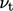is still required to solve the ensemble-averaged momentum equation, e.g. Eq. (6.26 ). The discussion in Sec. 6.11 indicates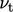is the product of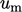and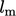, requiring two models to represent each scale. Since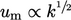,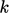can represent the speed scale, modelled by Eq. (6.28 ).

The turbulent dissipation rate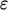matches the rate of transfer of kinetic energy down the energy cascade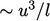, as discussed in Sec. 6.6 . This applies to all turbulent scales including the larger mixing length scales, so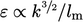. Substituting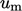and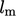expressions into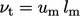yields turbulent viscosity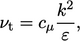(6.31)
where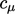is a constant. From empirical data,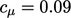, except within the viscous and buﬀer layers close to a wall, see Sec. 7.4 .

### Transport of turbulent dissipation rate

A model is now required for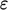, both to calculate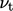by Eq. (6.31 ) and to provide the remaining unknown in Eq. (6.28 ). The model can be provided by a transport equation for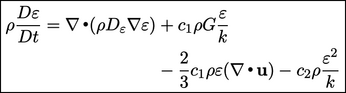(6.32)
where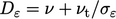is the combined molecular and turbulent diﬀusion with an adjustable coeﬃcient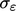, usually set to 1.3. The remaining coeﬃcients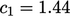and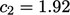are tuned to capture the behaviour of a range of ﬂows.

The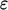-equation, Eq. (6.32 ), can be derived in terms of statistical properties, replacing high-order terms in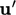by models with coeﬃcients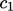,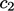.18

Alternatively, it can be obtained by multiplying the principal variable,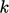or, in each term of Eq. (6.28 ) by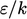and introducing coeﬃcients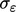,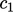and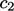.

The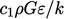term in Eq. (6.32) causes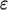to increase with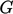. This is logical since the generated turbulence moves down the energy cascade, so ultimately aﬀects the rate of dissipation.

The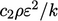term is justiﬁed by considering the free decay of turbulence. If the ﬂuid stops moving (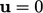) and turbulence is no longer generated (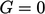), then (assuming constant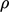and ignoring diﬀusion) Eq. (6.28 ) and Eq. (6.32) reduce to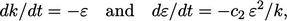(6.33)
respectively. Integrating the combined equations yields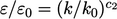where the “0” subscript indicates initial values.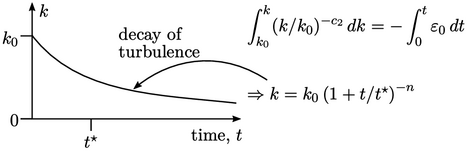Further integration yields a decay in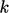over time to the power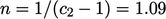and a timescale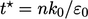, which is a reasonable approximation to real behaviour.

18B. I. Davydov, Statistical dynamics of an incompressible turbulent ﬂuid, Dokl. Akad. Nauk SSSR 136, 1961.

Notes on CFD: General Principles - 6.14 Turbulent dissipation rate